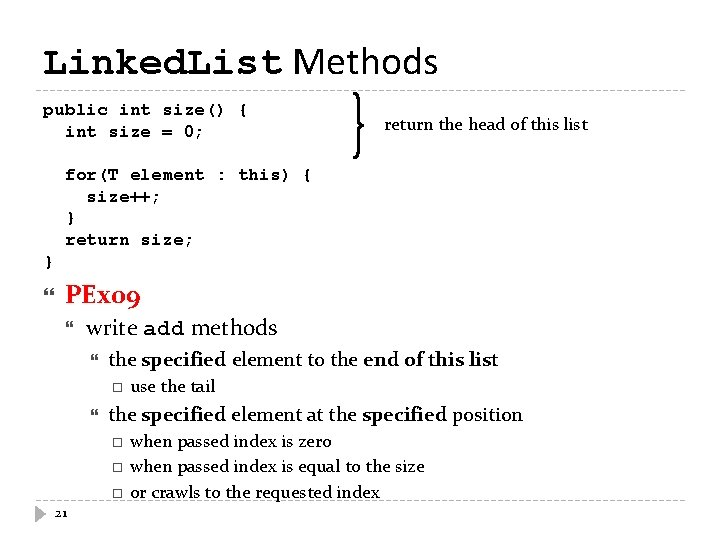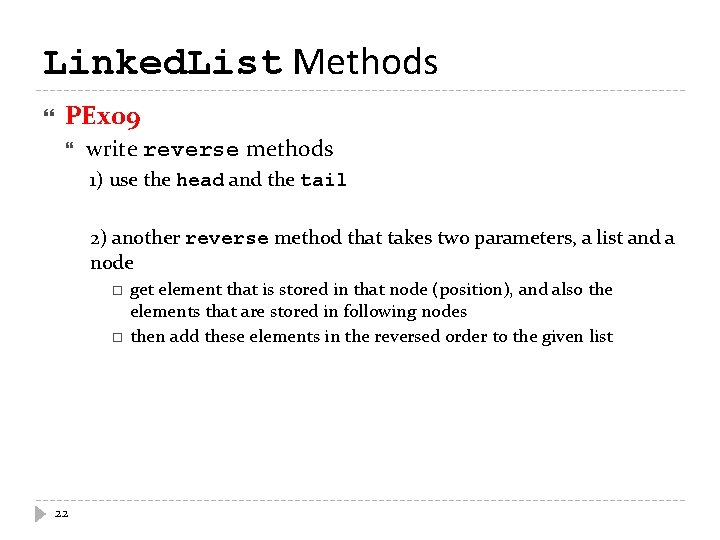• Slides: 22Linked Lists – Revisited a common way of storing arrays of data can insert or remove elements without reallocating or reorganizing of the entire structure allows insertion and removal of nodes at any point in the list we do not have to specify a fixed size many data structures often implemented using the concept of linked list 2 stacks, queues, associative arrays, etc.Anatomy of a Linked List a linked list consists of: a sequence of nodes my. List a b c d each node contains a value (data) and a link (pointer or reference) to some other node the last node contains a null link the list may have a header 3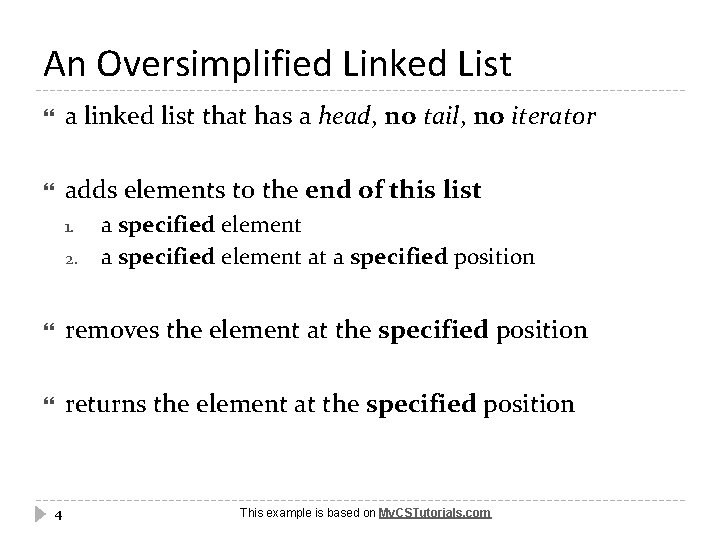An Oversimplified Linked List a linked list that has a head, no tail, no iterator adds elements to the end of this list 1. 2. a specified element at a specified position removes the element at the specified position returns the element at the specified position 4 This example is based on My. CSTutorials. comNode Class a a node must contain: 1. 2. reference to the next node in the chain (or null if there isn't one) data carried by this node, which could be of any type public class Node { Node next; Object data; // constructor section 5Node Constructors two constructors: 1. to create a node with no next node public Node(Object data) { this. next = null; this. data = data; } 1. to create a node with the given data and next node public Node(Object data, Node next) { this. next = next; this. data = data; } 6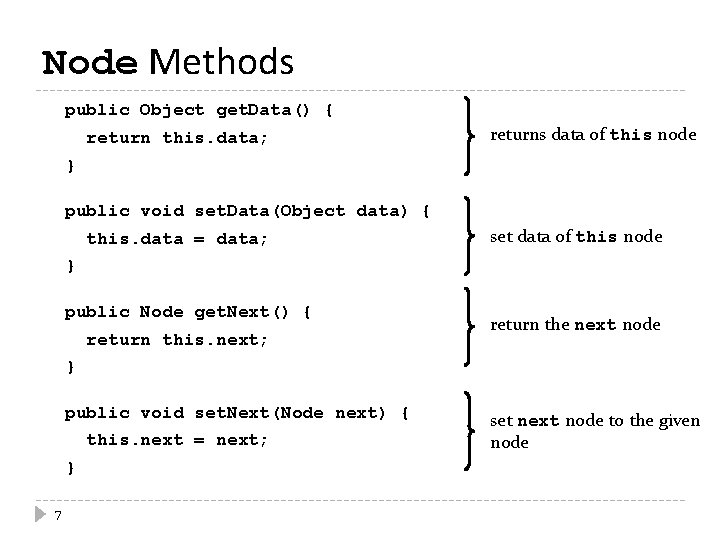Node Methods public Object get. Data() { return this. data; returns data of this node } public void set. Data(Object data) { this. data = data; set data of this node } public Node get. Next() { return this. next; return the next node } public void set. Next(Node next) { this. next = next; } 7 set next node to the given nodeLinked. List Class we need a reference to the head node public class Linked. List { private Node head; private int list. Count; // constructor section // method section } 8Linked. List Constructor public Linked. List() { head = new Node(null); list. Count = 0; } creates an empty list 9 reference to the head node is set to a new node with no data list. Count keeps count of the number of elements in the listLinked. List add Method public void add(Object data) { Node temp = new Node(data); Node current = head; while(current. get. Next() != null) { current = current. get. Next(); } start at the head node, then crawl to the end of the list current. set. Next(temp); list. Count++; } the last node now referring to the new node that we have just added adds the specified element to the end of this list 10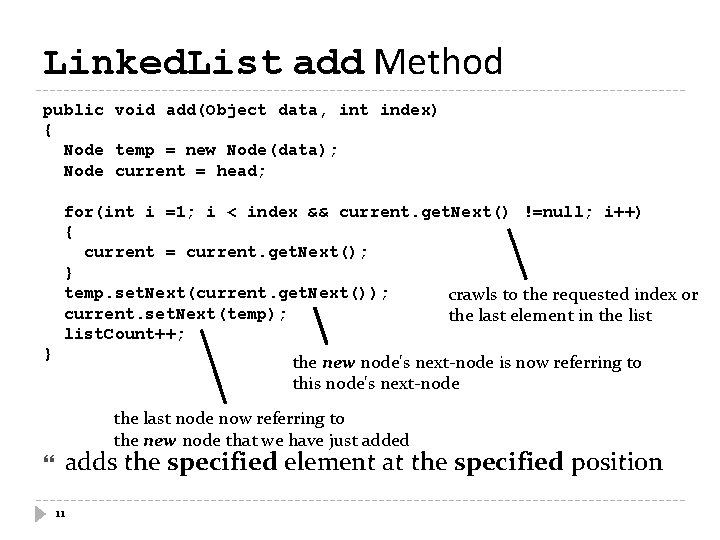Linked. List add Method public void add(Object data, int index) { Node temp = new Node(data); Node current = head; for(int i =1; i < index && current. get. Next() !=null; i++) { current = current. get. Next(); } temp. set. Next(current. get. Next()); crawls to the requested index or current. set. Next(temp); the last element in the list. Count++; } the new node's next-node is now referring to this node's next-node the last node now referring to the new node that we have just added adds the specified element at the specified position 11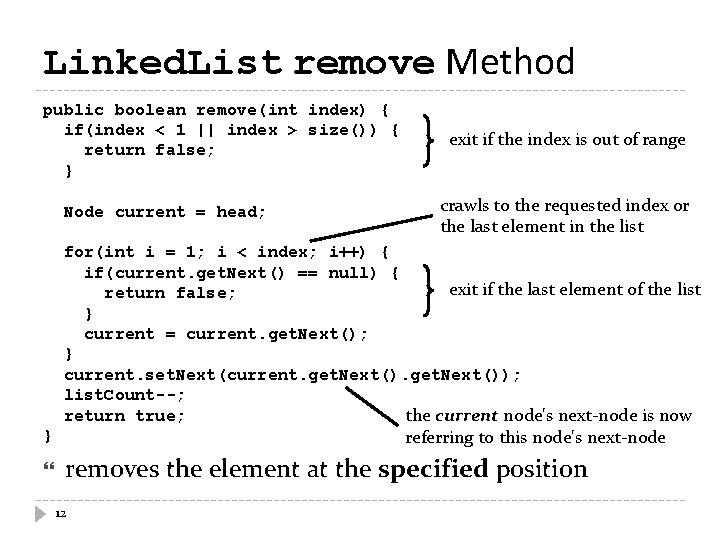Linked. List remove Method public boolean remove(int index) { if(index < 1 || index > size()) { return false; } Node current = head; exit if the index is out of range crawls to the requested index or the last element in the list for(int i = 1; i < index; i++) { if(current. get. Next() == null) { exit if the last element of the list return false; } current = current. get. Next(); } current. set. Next(current. get. Next()); list. Count--; return true; the current node's next-node is now referring to this node's next-node } removes the element at the specified position 12Linked. List get Method public Object get(int index) { if(index <= 0) return null; index must be at least 1 Node current = head. get. Next(); for(int i = 1; i < index; i++) { if(current. get. Next() == null) { return null; } exit if the last element of the list current = current. get. Next(); } return current. get. Data(); } returns the element at the specified position 13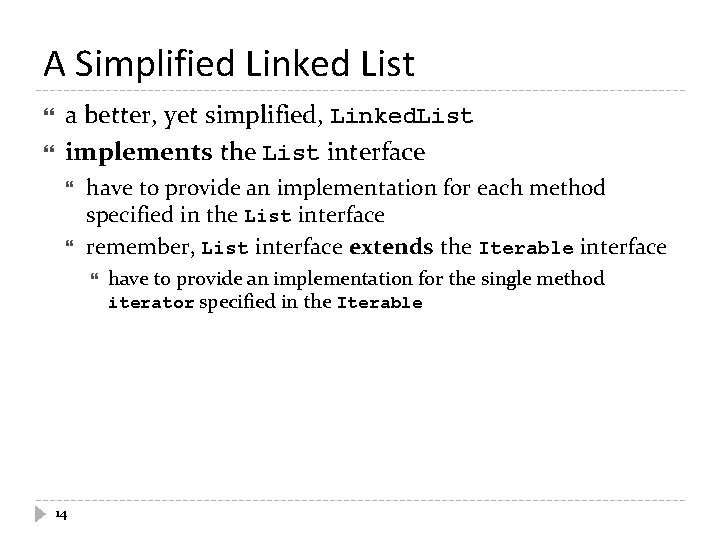A Simplified Linked List a better, yet simplified, Linked. List implements the List interface have to provide an implementation for each method specified in the List interface remember, List interface extends the Iterable interface 14 have to provide an implementation for the single method iterator specified in the IterableNode Class a a node must contain: 1. 2. reference to the next node in the chain (or null if there isn't one) data carried by this node, which could be of any type public class Node<T> { private T element; private Node<T> next; // constructor section 15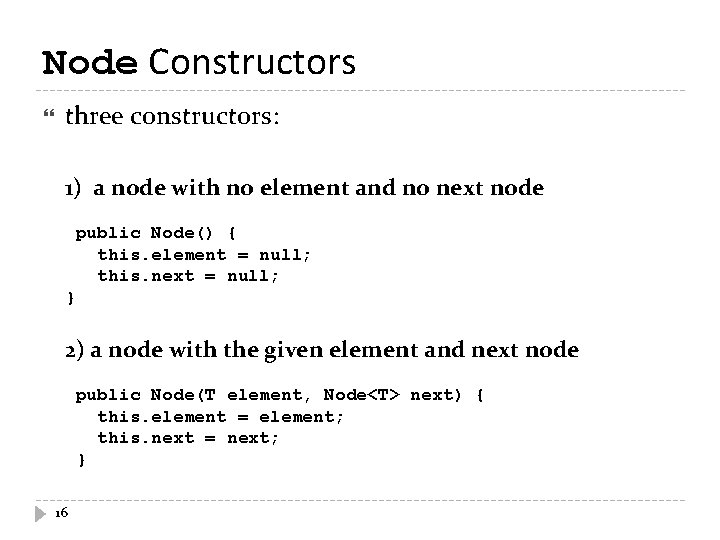Node Constructors three constructors: 1) a node with no element and no next node public Node() { this. element = null; this. next = null; } 2) a node with the given element and next node public Node(T element, Node<T> next) { this. element = element; this. next = next; } 16Node Constructors 3) creates a deep copy of the given node. public Node(Node<T> copy) { this. element = copy. element; if (copy. next == null) { if an empty list this. next = null; } else { this. next = new Node<T>(copy. next); } } 17Node Methods public T get. Element() { return this. element; returns data of this node } public Node<T> get. Next() { return this. next; return the next node } public void set. Next(Node<T> next) { this. next = next; } 18 set next node to the given node